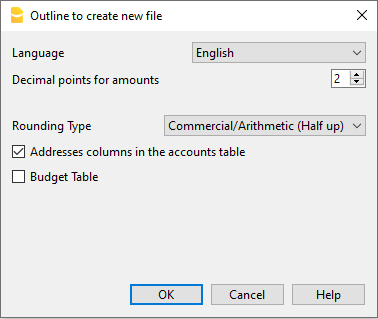# Outline to create new file

Documentation •## Language

The language used for the columns headers of the various tables.

## Decimals and rounding

#### Decimal points for amounts

In order to avoid accounting differences, the number of decimal points for the amounts is fixed for each file. Two decimal points are normally used, but there are currencies where decimal points are not required so 0 needs to be inserted.

For other currencies that use multiple decimals such as the Tunisian Dinar or crypto currencies such as Bitcoin (9 decimal places), Ethereum (18 decimal places) the appropriate number must be indicated.
Accounting can be managed with amounts of up to 27 decimal places.

#### Decimal points for amounts in foreign currency

When using a multi-currency accounting, the number of decimals for the amounts in foreign currency must be defined. For example, if the accounting is in EUR and you are managing crypto currencies such as Ethereum, you must enter 18.
Generally, the number of decimals must not be less than the number of decimals in the base currency.

#### Rounding type

The rounding is applied to amounts with decimals that go beyond the set number of decimals.
If 2 decimals are set, this is the type used to round the digits over two decimals.
Depending on the methods used, the following amounts: 1.005; 1,015; 1,025; 1,035 will be rounded in a different way.

• Commercial/Arithmetic (Half up) rounding to the next whole number: 1.01; 1.02; 1.03; 1.04.. In accounting, this is most commonly used.
• Banking (Half average) rounding up or down towards the nearest even number: 1.01; 1.02; 1.03; 1.04.

## Functionalities to be included

#### Address fields in accounts table

When this option is activated, the Address view is created in the Accounts table. In this view, the user can enter the addresses of clients, suppliers and members.

#### Budget Table

When this option is activated a Budget Table is created, where the user can enter his budget transactions.

Accounting files, in different languages can thus be created, and with different decimal points. In order to change the language or the decimal points once the accounting file has been created, the accounting type must be converted via Tools  → Convert to new file  from the menu.

help_id
DlgBanCartellaImpostazioniNuova